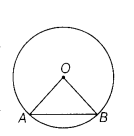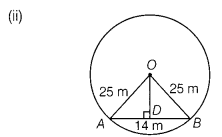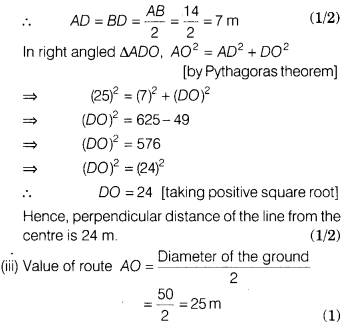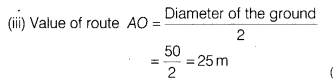# In VNSD school, there is a ground, where the students are playing. T

In VNSD school, there is a ground, where the students are playing. The shape of the ground is circular with diameter of 50 m. One line segment is drawn in the field of length 14 m. [use, n =3.14]
(i) Determine the perimeter of the circle.
(ii) Determine the perpendicular distance from the centre to this line segment.
(iii) Suppose, one student Neeraj is standing at the end point A of a line. He wants to reach the centre of a circle O in shortest time. So, he consider by the route AO. Find the’value of route AO.
(iv) Which quality is shown by the Neeraj?Given, diameter of circle, d = 50 m
∴ Radius of circle, r =50/2 =25m
(i) Now, perimeter of circle=2πr
=2 × 3.14 × 25 = 157mIn the given figure, if we draw a perpendicular line from centre of circle to the line segment, then it bisects the line segment.Hence, perpendicular distance of the line from the centre is 24 m.(iv) Neeraj shows the quality of intelligency and smartness.